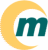s i s t e m a   o p e r a c i o n a l   m a g n u x   l i n u x ~/ · documentaçăo · suporte · sobre

Next Previous Contents

## 9. Consequences

What does all of this mean? For Linux users only one thing: that they must make sure that LILO and `fdisk` use the right geometry where `right' is defined for `fdisk` as the geometry used by the other operating systems on the same disk, and for LILO as the geometry that will enable successful interaction with the BIOS at boot time. (Usually these two coincide.)

How does `fdisk` know about the geometry? It asks the kernel, using the `HDIO_GETGEO` ioctl. But the user can override the geometry interactively or on the command line.

How does LILO know about the geometry? It asks the kernel, using the `HDIO_GETGEO` ioctl. But the user can override the geometry using the ``disk=`' option in `/etc/lilo.conf` (see lilo.conf(5)). One may also give the `linear` option to LILO, and it will store LBA addresses instead of CHS addresses in its map file, and find out of the geometry to use at boot time (by using INT 13 Function 8 to ask for the drive geometry).

How does the kernel know what to answer? Well, first of all, the user may have specified an explicit geometry with a ``hda=`cyls`,`heads`,`secs' kernel command line option (see bootparam(7)), perhaps by hand, or by asking the boot loader to supply such an option to the kernel. For example, one can tell LILO to supply such an option by adding an ``append = "hda=`cyls`,`heads`,`secs`"`' line in `/etc/lilo.conf` (see lilo.conf(5)). And otherwise the kernel will guess, possibly using values obtained from the BIOS or the hardware.

It is possible (since Linux 2.1.79) to change the kernel's ideas about the geometry by using the `/proc` filesystem. For example

``` # sfdisk -g /dev/hdc /dev/hdc: 4441 cylinders, 255 heads, 63 sectors/track # cd /proc/ide/ide1/hdc # echo bios_cyl:17418 bios_head:128 bios_sect:32 > settings # sfdisk -g /dev/hdc /dev/hdc: 17418 cylinders, 128 heads, 32 sectors/track # ```
This is especially useful if you need so many boot parameters that you overflow LILO's (very limited) command line length.

How does the BIOS know about the geometry? The user may have specified it in the CMOS setup. Or the geometry is read from the disk, and possibly translated as specified in the setup. In the case of SCSI disks, where no geometry exists, the geometry that the BIOS has to invent can often be specified by jumpers or setup options. (For example, Adaptec controllers have the possibility to choose between the usual H=64, S=32 and the `extended translation' H=255, S=63.) Sometimes the BIOS reads the partition table to see with what geometry the disk was last partitioned - it will assume that a valid partition table is present when the 55aa signature is present. This is good, in that it allows moving disks to a different machine. But having the BIOS behaviour depend on the disk contents also causes strange problems. (For example, it has been reported that a 2.5 GB disk was seen as having 528 MB because the BIOS read the partition table and concluded that it should use untranslated CHS. Another effect is found in the report that unpartitioned disks were slower than partitioned ones, because the BIOS tested 32-bit mode by reading the MBR and seeing whether it correctly got the 55aa signature.)

How does the disk know about the geometry? Well, the manufacturer invents a geometry that multiplies out to approximately the right capacity. Many disks have jumpers that change the reported geometry, in order to avoid BIOS bugs. For example, all IBM disks allow the user to choose between 15 and 16 heads, and many manufacturers add jumpers to make the disk seem smaller than 2.1 GB or 33.8 GB. See also below. Sometimes there are utilities that change the disk firmware.

## 9.1 Computing LILO parameters

Sometimes it is useful to force a certain geometry by adding ``hda=`cyls`,`heads`,`secs' on the kernel command line. Almost always one wants secs=63, and the purpose of adding this is to specify heads. (Reasonable values today are heads=16 and heads=255.) What should one specify for cyls? Precisely that number that will give the right total capacity of C*H*S sectors. For example, for a drive with 71346240 sectors (36529274880 bytes) one would compute C as 71346240/(255*63)=4441 (for example using the program `bc`), and give boot parameter `hdc=4441,255,63`. How does one know the right total capacity? For example,

``` # hdparm -g /dev/hdc | grep sectors geometry = 4441/255/63, sectors = 71346240, start = 0 # hdparm -i /dev/hdc | grep LBAsects CurCHS=16383/16/63, CurSects=16514064, LBA=yes, LBAsects=71346240 ```
gives two ways of finding the total number of sectors 71346240. The kernel output
``` # dmesg | grep hdc ... hdc: Maxtor 93652U8, 34837MB w/2048kB Cache, CHS=70780/16/63 hdc: [PTBL] [4441/255/63] hdc1 hdc2 hdc3! hdc4 < hdc5 > ... ```
tells us about (at least) 34837*2048=71346176 and about (at least) 70780*16*63=71346240 sectors. In this case the second value happens to be precisely correct, but in general both may be rounded down. This is a good way to approximate the disk size when `hdparm` is unavailable. Never give a too large value for cyls! In the case of SCSI disks the precise number of sectors is given in the kernel boot messages:
``` SCSI device sda: hdwr sector= 512 bytes. Sectors= 17755792 [8669 MB] [8.7 GB] ```
(and MB, GB are rounded, not rounded down, and `binary').

Next Previous Contents